Register
Support Center

Visit our Support Center. It's the easiest way to access all the crypto information needed.

FameEX provides a user-friendly, secure, reliable, and excellent crypto trading experience. Download our mobile APP and Trade your Bitcoin anywhere and whenever you want!Go trade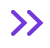Support Center
Futures
How to Calculate Liquidation Price

# How to Calculate Liquidation Price

2021/09/18 18:26:15

U-Futures trading is a contract that guarantees a position. Traders need to keep a certain percentage of their funds as a maintenance margin. If the user’s margin is lower than the maintenance margin, it will trigger the auto deleveraging system or liquidation.

Our system will calculate the estimated liquidation price based on our traders’ both long and short positions and funding when the trader opens the position. The estimated liquidation price can be a reference of risk for long and short positions. There may be a slight difference in price between the estimated liquidation price and the real-time liquidation price due to the volatile market.

For example,

If the trader has 100 USDT in the account, the mark price is 500 USDT and the entry price is 501 USDT, then the maintenance margin rate would be 0.5% and the Transaction fee rate is 0.01%.

Scenario 1: If the trader is under the cross margin mode and opens a long position with leverage of 20X, how to calculate the liquidation price?

1. Transaction Fee= Position Value X Transaction Fee Rate = 501*0.01%= 0.0501 USDT

2. Account Balance = Account Asset - Transaction Fee of Cross Margin = 100-0.051USDT= 99.9499 USDT

3. The Estimated Liquidation Price (ELP) of Cross Mode

= [Account Balance - Isolated Margin + (ELP - Average Position Price) X Size of Long Position + (Average Position Price - ELP) X Size of Short Position + Unrealized PnL - Maintenance Margin of Cross Contract] / (Size of Long Position X ELP + Size of Short Position X ELP ) = Maintenance Margin Rate

With this information and by inputting the appropriate information in the formula we can see that,

[99.9499 + (ELP-501) *1] / ELP = 0.5 %

ELP= 403.07 USDT

Scenario 2: If the trader is under the isolated margin mode and opens a 1 ETH long position with a leverage of 20X, how to calculate the liquidation price?

1. Transaction Fee= Position Value X Transaction Fee Rate = 501*0.01%= 0.0501 USDT

2. Maintenance margin of Isolated Position

= (Position Value X Size / LeverageX) - Transaction Fee

= (501*1/20)-0.0501

=24.9999 USDT

3. The Estimated Liquidation Price (ELP)

=[Isolated Margin + (ELP - Average Position Price) X Size of Long Position + (Average Position Price - ELP) X Size of Short Position] /  (Size of Long Position X ELP + Size of Short Position X ELP) = Maintenance Margin Rate

With this information and by inputting the appropriate information in the formula we can see that,

[24.9999 + (ELP-501)* 1] / ELP = 0.5 %

ELP=478.39 USDT

•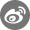•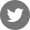•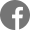•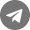•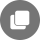Other articles
Related Articles
Bug reports: Safe@mail.fameex.info